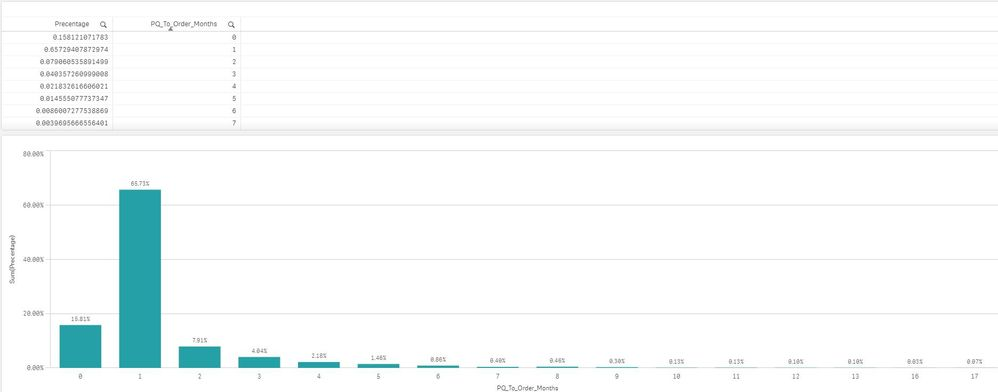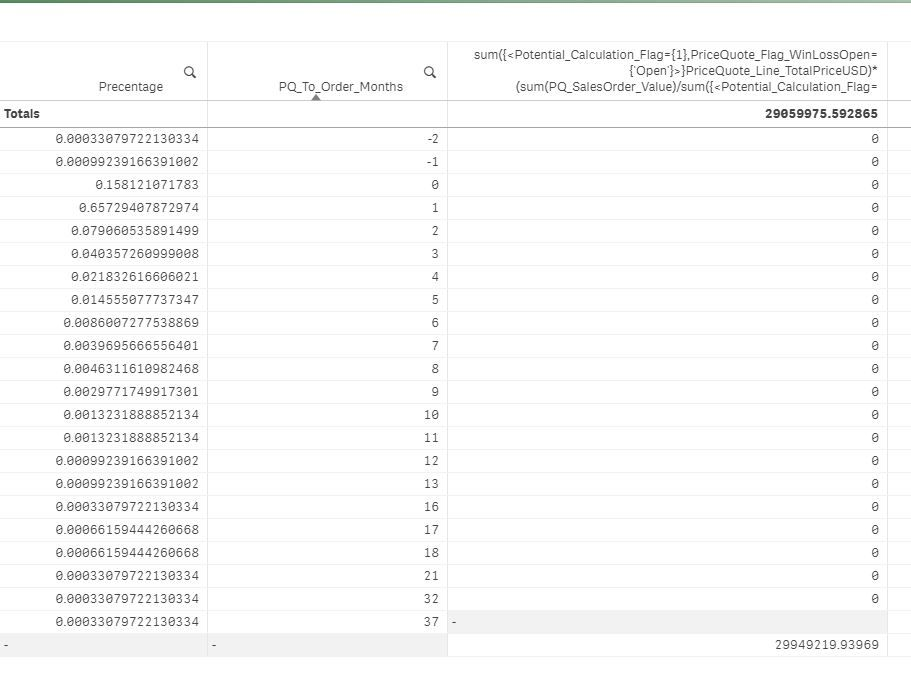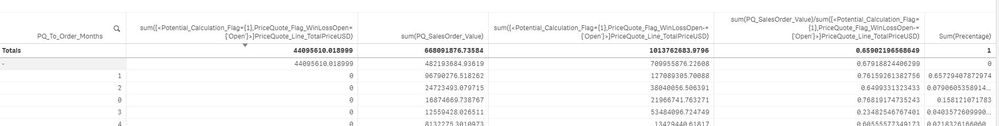# New to Qlik Sense

If you’re new to Qlik Sense, start with this Discussion Board and get up-to-speed quickly.

cancel
Showing results for
Did you mean:Creator

## multiply a measure outcome with other data

Hi,

a little bit of background:

i have an object-Price Quotes (PQ), some of them are regular and some of them are with "steps" - few lines for the same part with different prices - and the customer will usually order only one of the lines.
(qty = 1 - price =x / qty=3 - price=y /...)
the first measure i've created is the potential of the PQ's (sum of all regular PQs + the maximum potential of the stepped ones)

so i've created a flag in the script weather a line should be calculated as potential or not - "Potential_Calculation_Flag"
and i've calculated the potential for open quotes:

sum({<Potential_Calculation_Flag={1},PriceQuote_Flag_WinLossOpen={'Open'}>}PriceQuote_Line_TotalPriceUSD)

after the potentail was found, i had to find the realization percentage so i calculated the linked orders value and divided by the potentail

percentage:
sum(PQ_SalesOrder_Value)/sum({<Potential_Calculation_Flag={1},PriceQuote_Flag_WinLossOpen-={'Open'}>}PriceQuote_Line_TotalPriceUSD)

Sum:
sum({<Potential_Calculation_Flag={1},PriceQuote_Flag_WinLossOpen={'Open'}>}PriceQuote_Line_TotalPriceUSD)*(sum(PQ_SalesOrder_Value)/sum({<Potential_Calculation_Flag={1},PriceQuote_Flag_WinLossOpen-={'Open'}>}PriceQuote_Line_TotalPriceUSD))

now my question is:

i went and check how long did it take to win a PQ (Order Date - PQ Date), and created a percentage for each month
(PriceQuote_SalesOrderDate-PriceQuote_Date)/30 as PQ_To_Order_Months_tmp

now my question is:

i want to multiply the sum of realization with the percentage and get the expected realization for each coming month

sum({<Potential_Calculation_Flag={1},PriceQuote_Flag_WinLossOpen={'Open'}>}PriceQuote_Line_TotalPriceUSD)*(sum(PQ_SalesOrder_Value)/sum({<Potential_Calculation_Flag={1},PriceQuote_Flag_WinLossOpen-={'Open'}>}PriceQuote_Line_TotalPriceUSD))
*
Sum(Percentage)

but this doesn't seem to work, not in a table and not in a chart (
how can i do it?the monthly percentage is linked to the Price quotes with the PQ_To_Order_Months

i've tried to do it with and without a joinany ideas?

Many Thanks,

Roi

Labels (4)

• ### Set Analysis

1 Solution

Accepted SolutionsCreator
Author

Issue solved.

i've disconnected the PQ_To_Order_Months from the PQ table and created it as a stand alone table

with Months | Percentage

Thanks! 🙂

4 RepliesCreator
Author

anyone? 😏Specialist II

Hard for me to follow without actually working in your app.

Can you make 3 seperate expressions in a table with only PQ_To_Order_Months as dimension, and see what the results are for each piece:

1)

sum({<Potential_Calculation_Flag={1},PriceQuote_Flag_WinLossOpen={'Open'}>}PriceQuote_Line_TotalPriceUSD)

2)

(sum(PQ_SalesOrder_Value)/sum({<Potential_Calculation_Flag={1},PriceQuote_Flag_WinLossOpen-={'Open'}>}PriceQuote_Line_TotalPriceUSD))

3)
Sum(Percentage)Creator
Author

@stevejoyce  Thank you for your replay!

and thank you for setting my mind straight, first thing of course is it separate the measures,

once i did it, i realized that the first expression is calculated only for Open PQ's while the PQ_To_Order_Months  is for closed PQ's    😕

how do i combine the two?

i need to get the total realization (that can be changed according to filters) and multiply it by the percentage for each month that came from the closed PQ'sCreator
Author

Issue solved.

i've disconnected the PQ_To_Order_Months from the PQ table and created it as a stand alone table

with Months | Percentage

Thanks! 🙂Tags
Community Browser